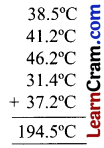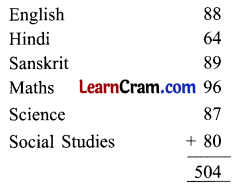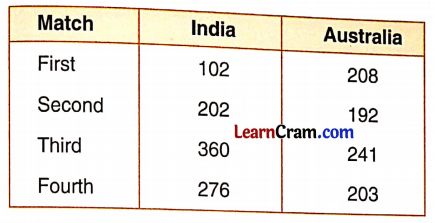# DAV Class 5 Maths Chapter 10 Worksheet 2 Solutions

The DAV Books Solutions Class 5 Maths and DAV Class 5 Maths Chapter 10 Worksheet 2 Solutions of Averages offer comprehensive answers to textbook questions.

## DAV Class 5 Maths Ch 10 Worksheet 2 Solutions

Question 1.
Solve the following word problems.
(a) The ages of the six children chosen for project work are 13 years, 16 years, 14 years, 17 years, 16 years and 17 years respectively. What is the average age of a child on the team?
Solution:
Total age of six childrenAverage age of a child in the team = 90 ÷ 6 = 15 years
15 years is the average age of a child.(b) Sachin scored 98, 25, 105, 62, and 65 runs in five matches. What was his average score in a match?
Solution:
The total run Sachin scoredAverage score of Sachin = 355 ÷ 5 = 71
71 runs is his average score.

(c) Rama earned ₹ 65, ₹ 82, ₹ 79, ₹ 75, ₹ 90 and ₹ 83 in six days. Find his average earning in a day.
Solution:
Total earnings of RamaAverage earning in a day = 474 ÷ 6 = ₹ 79
₹ 79 is the average earnings in a day.

(d) The temperature of a city in five days was 38.5°C, 41.2°C, 46.2°C, 31.4°C and 37.2°C. What was the average daily temperature?
Solution:
The total temperature of a cityAverage daily temperature = 194.5 ÷ 5 = 38.9°C
38.9°C was the average daily temperature.

(e) Bharat scored the following marks in six subjects.Find his average score in a subject.
Solution:
The total score of Bharat in six subjectsAverage score in a subject= 504 ÷ 6 = 84
84 is the average score in a subject.(f) In an office, 20 employees get a salary of ₹ 1,250 each, and 10 employees get a salary of ₹ 2,000 each. Find the average salary of an employee.
Solution:
Total Salary of employees = 20 × 1,250 + 10 × 2,000
= 25,000 + 20,000
= ₹ 45,000
Average salary of an employee = $$\frac{45000}{20+10}$$
= $$\frac{45000}{30}$$
= ₹ 1500
The average salary of an employee is ₹ 1500.

(g) In a series of four cricket matches, the runs scored by India and Australia are given below:(i) What is the average score of India in a match?
(ii) What is the average score of Australia in a match?
(iii) Which team performed better?
Solution:
(i) Total run scored by India in series = 102 + 202 + 360 + 276 = 940
Average score of India = $$\frac{940}{4}$$ = 235
235 runs is the average score of India in a match.

(ii) Total run scored by Australia in series = 208 + 192 + 241 + 203 = 844
Average score of Australia = $$\frac{844}{4}$$ = 211
211 runs is the average score of Australia in a match.

(iii) As the average score of India is better than Australia so India performed better.

### DAV Class 5 Maths Chapter 10 Value Based Questions

Books are our best friends. Reading books not only increases our knowledge but also keeps us refreshed. But some children cannot afford to buy books. So the students of a school decided to donate their old storybooks to the needy children in a locality. Classes VA, VB, VC, VD, and VE donated 21 books, 18 books, 23 books, 26 books, and 32 books respectively. These books were collected and then distributed in the locality.

Question 1.
What is the average number of storybooks donated by a class?
Solution:
Total books donated by students = 21 + 18 + 23 + 32 + 26 = 120
Average number of books donated by a class = $$\frac{120}{5}$$ = 24 books
24 books is the average number of storybooks donated by a class.Question 2.
What are the advantages of a class library?
Solution:
Class libraries have different types of storybooks donated by each child. So it has many advantages like-

• Different types of storybooks are present there.
• Children make a rotation in class (of these books) and share the story of these books.
• Wastage of books in the house of students is reduced.
• When the rotation is completed, we can donate these books to poor or needy children.

Question 3.
How many books have you donated to your class library?
Solution:
Do it yourself.

Word Problem

Example.
The weight of four children in the medical test is given below:
Aman – 36.2 kg
Nina – 34.8 kg
Prateek – 40.3 kg
Annu – 42.3 kg
(a) Find the average weight.
(b) Flow many children have weight less than the average?
(c) Name the children whose weight is above average.
Solution:
(a) Total weight of childrenThe average weight of one child = 153.6 kg ÷ 4 = 38.4 kg.
(b) Two children have their weights less than the average weight.
(c) The weight of Prateek and Annu is above average.